# Saxon Algebra 1: Quadratic Equations Chapter Exam

Exam Instructions:

Choose your answers to the questions and click 'Next' to see the next set of questions. You can skip questions if you would like and come back to them later with the yellow "Go To First Skipped Question" button. When you have completed the practice exam, a green submit button will appear. Click it to see your results. Good luck!

### Page 1

#### Question 3 3. When completing the square on the quadratic equation listed below, how is c calculated?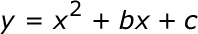### Page 2

#### Question 7 7. If the equation below told you a ball was 'h' feet in the air after 't' seconds, what would be the first step to finding out when it hit the ground?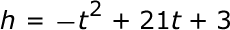### Page 3

#### Question 11 11. Find the roots of the equation below.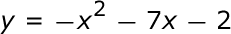#### Question 12 12. In order to complete the square on the following equation, what value would you add to both sides?#### Question 13 13. What is the b-value of the quadratic equation below?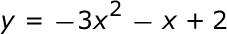### Page 4

#### Question 17 17. Solve the equation below.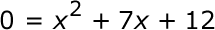#### Question 18 18. Solve the equation below.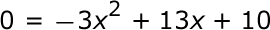#### Question 20 20. Use the quadratic formula to find the solutions to the equation below.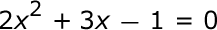#### Saxon Algebra 1: Quadratic Equations Chapter Exam Instructions

Choose your answers to the questions and click 'Next' to see the next set of questions. You can skip questions if you would like and come back to them later with the yellow "Go To First Skipped Question" button. When you have completed the practice exam, a green submit button will appear. Click it to see your results. Good luck!

Support